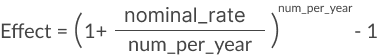# Effect

The Effect function returns the effective annual interest rate.

Lending institutions advertise the nominal rate of their loans. The nominal interest rate does not take into account the effect of compounding. The effective interest rate takes the compounding period into account, and it is a more accurate measure of interest charges.

Effect is part of the set of financial functions that Sigma supports.

## Syntax

`Effect(nominal_rate, num_per_year)`

The Effect function has the following arguments:

nominal_rate
Required.
Nominal interest rate
To use 7%, use the value 0.07.
num_per_year
Required.
Number of compounding periods per year

The general formula for the Effect function is:## Examples

`Effect(.0678, 12)`

The effective annual interest rate for an effective rate of 6.78%, paid 12 times a year (monthly). This example returns 7.00%.

`Nominal(0.677, 26)`

The nominal annual interest rate for an effective rate of 6.77%, paid 26 times a year (bi-weekly). This example returns 7.00%.

`Effect(0.1, [Number of Periods]`

Explore how the the effective annual interest rate changes when the nominal interest rate is 10% applies to investments with a different number of periods.